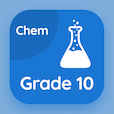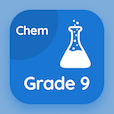SAT Prep Courses

SAT Chemistry Prep Tests

SAT Chemistry Tests

The e-Book Finding The Order of Reaction Multiple Choice Questions (MCQ Quiz), Finding The Order of Reaction quiz answers PDF to learn online courses, sat chemistry tests. Study Rates of Reactions Multiple Choice Questions and Answers (MCQs), Finding The Order of Reaction quiz questions for high school entrance exam. The e-Book "Finding The Order of Reaction MCQ" App Download: catalysis, energy of activation, finding the order of reaction test prep for job placement test.

The MCQ "Arrhenius equation explains the effect of temperature on the" PDF, Finding The Order of Reaction App Download (Free) with rate of reaction, rate constant, average rate of reaction, and order of a reaction choices for high school entrance exam. Practice finding the order of reaction quiz questions, download Google eBook (Free Sample) for job placement test.

## SAT Chemistry: Finding The Order of Reaction MCQs

MCQ: Arrhenius equation explains the effect of temperature on the

A) Rate of reaction
B) Rate constant
C) Average rate of reaction
D) Order of a reaction

MCQ: The acid hydrolysis of an ester is an example of

A) First order
B) Pseudo first order
C) Second order
D) Third order

MCQ: Half-life of a reaction is inversely proportional to the

A) Initial concentration of reactants
B) Final concentration of products
C) Slow step of a reaction
D) Order of a reaction

MCQ: Dilatometry method is useful for the reaction that involves

A) Small volume changes in solution
B) Large volume changes in solution
C) Change in refractive indices
D) Ionic speciess

MCQ: The extent of rotation determines the concentration of substance

A) Optically active
B) Optically inactive
C) Chirality
D) Ionic character

### Practice Tests: SAT Chemistry Exam Prep

Download SAT Chemistry Quiz App, 10th Grade Chemistry MCQs App and 9th Grade Chemistry MCQ App for Android & iOS devices. These Apps include complete analytics of real time attempts with interactive assessments. Download Play Store & App Store Apps & Enjoy 100% functionality with subscriptions!